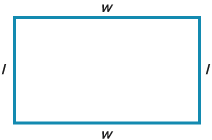# Polygons and Perimeters: Activity 3 of 3

## Directions

You already know how to calculate the perimeter of a rectangle, using the formula P = S1 + S2 + S3 + S4. But there's another formula you can use to do the same thing. Let's figure it out.

Rectangles have opposite sides that are equal in length.

## Math18_A3_Q1.pngQuestion 1 of 10